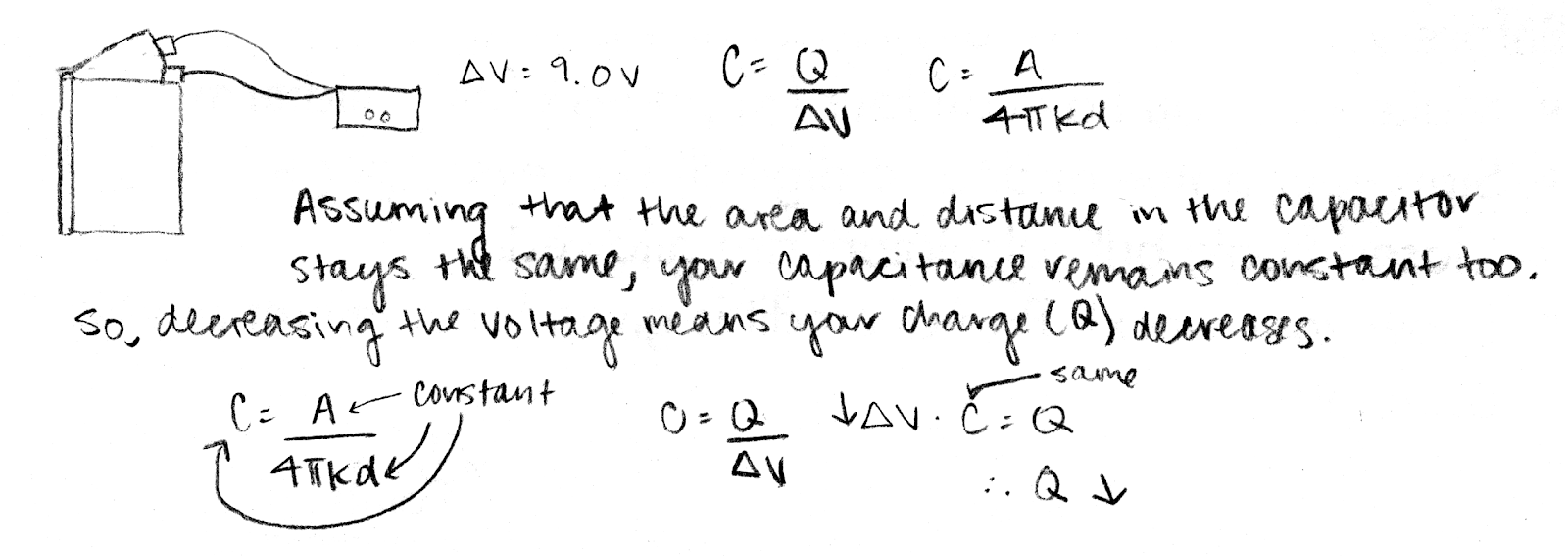## 20180505

### Physics midterm question: decreasing capacitor charge

Physics 205B Midterm 2, spring semester 2018
Cuesta College, San Luis Obispo, CA

A parallel-plate capacitor is constructed by placing two foil sheets between different pages in a phone book. The capacitor is then connected to a power source that slowly decreases the voltage applied from 9.0 V to 0 V. Discuss why the amount of charge stored in the capacitor must also decrease while this is happening. Explain your reasoning by using the properties of capacitors, charge, electric potential, and energy.

• p:
Correct. Discusses why the charge stored in the capacitor will decrease because:
1. the capacitance of the capacitor is constant, as it has been already constructed and thus its plate area and separation distance remain unchanged; and
2. as the applied voltage ΔV decreases, because the capacitance remains constant, then the charge stored must also decrease.
• r:
As (p), but argument indirectly, weakly, or only by definition supports the statement to be proven, or has minor inconsistencies or loopholes. Discusses (2), but assumes and does not explain (1) why capacitance is constant.
• t:
Nearly correct, but argument has conceptual errors, or is incomplete. Assumes and does not explain why capacitance is constant, and somehow argues from C = QV how Q is inversely related to ΔV; or discusses how decreasing ΔV causes a decreasing Q because EPE decreases (from EPE = (1/2)⋅Q⋅ΔV), but does not discuss why EPE is expected to decrease (from EPE = (1/2)⋅C(⋅ΔV)2, where capacitance is constant).
• v:
Limited relevant discussion of supporting evidence of at least some merit, but in an inconsistent or unclear manner. Some attempt at applying properties of capacitors, charge, electric potential, and energy.
• x:
Implementation/application of ideas, but credit given for effort rather than merit. No clear attempt at systematically applying properties of capacitors, charge, electric potential, and energy.
• y:
Irrelevant discussion/effectively blank.
• z:
Blank.
Sections 30882, 30883
Exam code: midterm02iFtW
p: 14 students
r: 8 students
t: 9 students
v: 2 students
x: 1 student
y: 0 students
z: 0 students

A sample "p" response (from student 4632):Another sample "p" response (from student 3693):